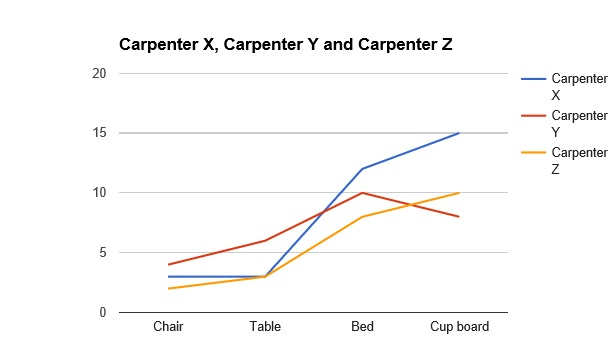## SBI PO Data Analysis Test 20

Instructions

Study the given graph carefully to answer the question that follow:

Number of days taken by three carpenters to finish making one piece each of four different items of furnitureQuestion 1

If Carpenter X and Carpenter Y were to make a chair together how many days would they take ?

Question 2

If Carpenter X, Y and Z were to make a table together, how many days would they take?

Question 3

What is the total number of days that Carpenter Z will take to make one piece each of all the four items together ?

Instructions

For the following questions answer them individually

Question 4

In how many different ways can the letters of the word STRESS be arranged ?

Question 5

The radius of a circular field is equal to the side of a square field whose perimeter is 784 feet. What is the area of the circular field?